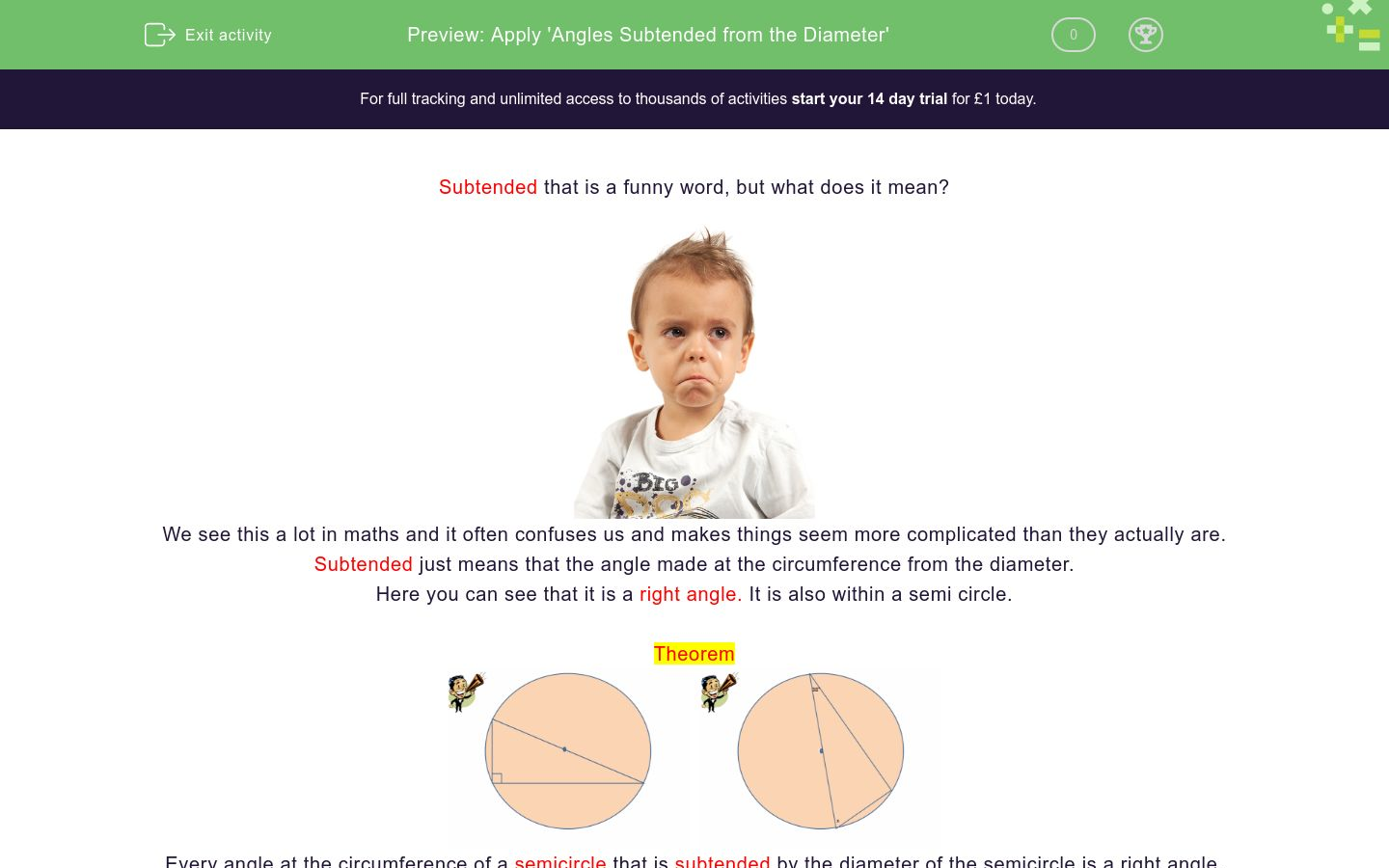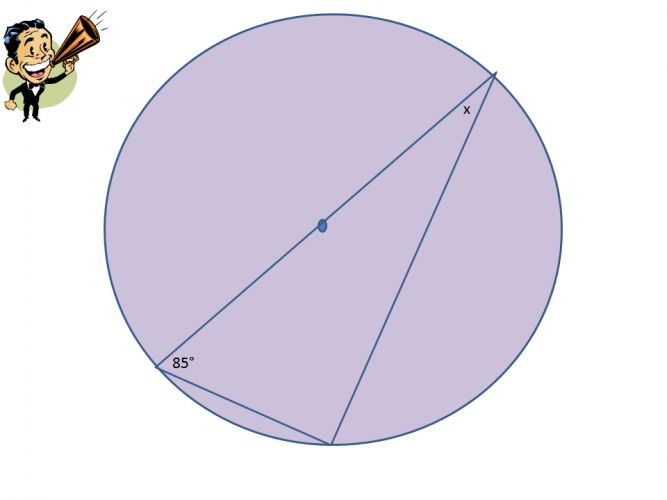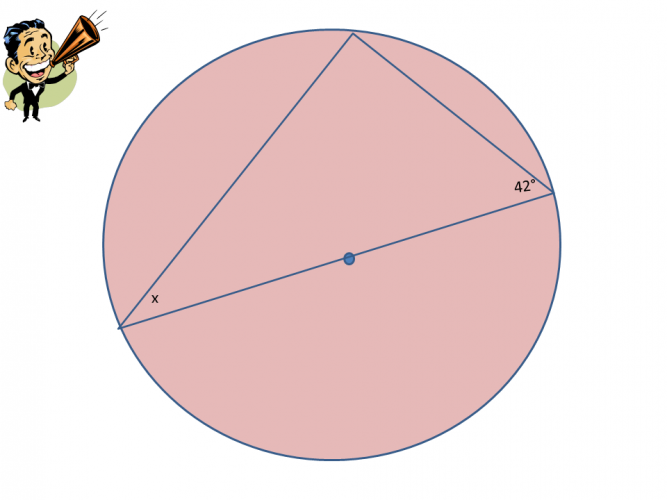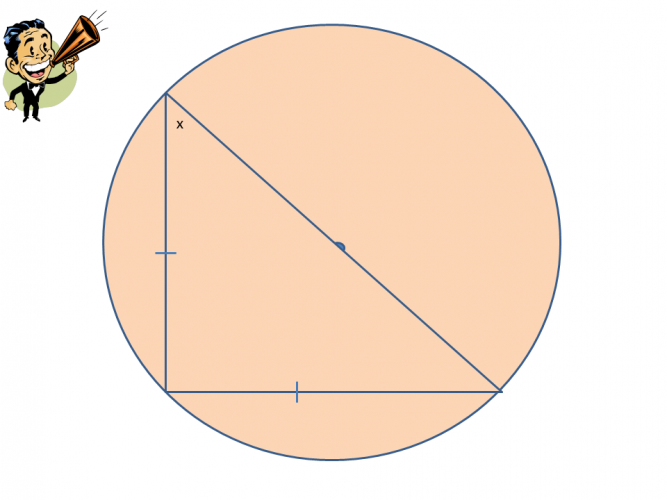# Apply 'Angles Subtended from the Diameter'

In this worksheet, students will identify and apply the circle theorem: 'angles subtended from the diameter'.Key stage:  KS 4

GCSE Subjects:   Maths

GCSE Boards:   OCR, AQA, Eduqas, Pearson Edexcel

Curriculum topic:   Basic Geometry, Geometry and Measures

Curriculum subtopic:   Circles, Properties and Constructions

Difficulty level:### QUESTION 1 of 10

Subtended that is a funny word, but what does it mean?We see this a lot in maths and it often confuses us and makes things seem more complicated than they actually are.

Subtended just means that the angle made at the circumference from the diameter.

Here you can see that it is a right angle. It is also within a semi circle.

TheoremEvery angle at the circumference of a semicircle that is subtended by the diameter of the semicircle is a right angle.If you draw a straight line from each end of the diameter to hit the circumference of the circle you will see a right angle.

So it is the angle made from the diameter at the circumference. That is all it means.

Example

In the example find x.

We know that angles in a triangle add up to 180°

We know that the one angle is a right angle 90°

The angle given is 38°

180 - 90 - 38 = 52°Have you noticed that circle theorems are just basic facts from our angle properties tool box?

Lets unlock it and use them.

Note: Circle theorem questions rarely show the right angle depicted by the square in the corner.What is the value of x?The value of x is

124°

34°

56°

65°What is the value of x.

 90° 85° 15° 5° Angle x isFind the value of x.True or false

 True False Angle x is 48°True False Angle x is 48°What is the value of x?What is the value of xHow do you find angle a?

Subtract 22° from 90°

Subtract 90° from 180°

Subtract 90° and 22° from 180°

• Question 1What is the value of x?

18
EDDIE SAYS
This is a nice angles in a triangle add up to 180° questions. We know it is a circle theorem because it is a right angle within a semi circle and we need to know the rule. 180 - 90 - 72 = 18°
• Question 2EDDIE SAYS
Did this throw you? I hope not. Remember quite often the right angle symbol isn't shown. But if you draw a line from each end of the diameter, it subtends (makes) the circumference at right angles.
• Question 3The value of x is

56°
EDDIE SAYS
I hope you are starting to see how easy these are. 180 - 90 - 34 = 56° We need to know the rule as the triangle is presented in the circle. Examiners just want to try and confuse us (but they can't can they?)
• Question 4What is the value of x.

 90° 85° 15° 5° Angle x is
EDDIE SAYS
Once you know the rule and can spot it, this really is quite simple. It is amazing how 180° and 90° are used in many areas of maths. 180 - 90 - 85 = 5°
• Question 5Find the value of x.

72
EDDIE SAYS
Are you getting to the stage where you could do these in your sleep? Keep dreaming of the rule. 180 - 90 - 18 = 72°
• Question 6True or false

 True False Angle x is 48°
EDDIE SAYS
Of course its true. 180 - 90 -42 = 48° Don't you just love it when they put simple maths into a problem that looks more complicated than it is. Circles aren't so pointless after all....
• Question 7EDDIE SAYS
And just when we thought it was going well. Ah wait, we can do this. We know that angle y is 90° according to the circle theorem. Good start. 180 - 90 = 90° 3x = 90° therefore x = 30° Great finish.
• Question 8What is the value of x?

45
EDDIE SAYS
We know that the angle subtended from the diameter is 90° The markings show that the triangle is isosceles so angle x and the other one are the same. 180-90 = 90 ÷ 2 = 45°
• Question 9What is the value of x

30
EDDIE SAYS
Okay so a little bit of thinking required here. The markings on the triangle show that it is equilateral. Therefore all angles are 60° Part of the right angle is therefore 60 and the remaining part is 30° The radii from the centre are the same length making the other triangle isosceles. Therefore x is also 30° as base angles in an isosceles triangle are the same. This is just looking at other angle properties through markings on the shape. This is why it is good to learn what notations and markings mean on shapes.
• Question 10How do you find angle a?

Subtract 90° and 22° from 180°
EDDIE SAYS
Once you know the formula, you know it. It just a case of spotting it within a circle theorem. The time you will need to have your wits about you is when more than once circle theorem is put into the same question.
---- OR ----